Online-Go.Com Player Statistics¶

[email protected] was nice enough to dump some information from their player database for me to analyze. The data available includes:

• Date of registration
• Ratings (ELO and dan/kyu)
• Rated games completed (wins, losses, draws)

I did some quick exploratory analysis, with plots below.

Setup (plotting, fetch data, convert to numpy arrays)¶

In :
# Setup, connect to database, fetch data
%pylab inline
from pylab import *
# from prettyplotlib import *
import numpy as np
import datetime

import matplotlib.pyplot as plt
plt.style.use('ggplot')

figsize(8,6)

# connect to the player database
import sqlite3
db = sqlite3.connect('playerinfo_dump-2016-05-06.db')
cursor = db.cursor()

# registrations are strings in YYYY-MM-DD HH:MM:SS... format
# last_active are in scientific notation in milliseconds since the epoch, but sometimes "None"
registered_dates, last_active, rating, games_count = \
zip(*cursor.execute('SELECT registered, last_active, rating, wins+losses+draws FROM pony').fetchall())

# Convert to numpy arrays
registered_dates = array([c[:10] for c in registered_dates], dtype='datetime64')
# convert to second since the epoch
last_active = array([(float(x) if x != "None" else -inf) for x in last_active]) / 1000
rating = array(rating)
games_count = array(games_count)

# Helper function for plotting time series nicely
import time
import datetime
import matplotlib.dates as mdates

def plot_time_series(dates, values):
# setup - see http://matplotlib.org/examples/api/date_demo.html
years    = mdates.YearLocator()   # every year
months   = mdates.MonthLocator()  # every month
yearsFmt = mdates.DateFormatter('%Y')

dates = dates.astype(datetime.datetime)

# plot user count vs time
plot_date(dates, values, '-k')
ax = gca()

ax.xaxis.set_major_locator(years)
ax.xaxis.set_major_formatter(yearsFmt)
ax.xaxis.set_minor_locator(months)

gcf().autofmt_xdate()

# useful for some plots where aliasing hides actual data
def jitter(data, lo=-1, hi=1):
return data + np.random.uniform(lo, hi, len(data))
Populating the interactive namespace from numpy and matplotlib
WARNING: pylab import has clobbered these variables: ['power', 'linalg', 'random', 'fft', 'info']
%matplotlib prevents importing * from pylab and numpy

Registration history¶

Online-Go.Com started as a turn-based server in 2006. Nova.gs started in July 2013 as a live or turn-based server, and merged with Online-Go.Com in October 2013. The data we have includes both sets of users.

In :
# registration dates are not sorted, due to the Nova/OGS merger
sorted_registered_dates = sort(registered_dates).astype(datetime.datetime)
plot_time_series(sorted_registered_dates, arange(len(sorted_registered_dates)))
title('Total registered users by date')
ylabel('# of registered users')
show()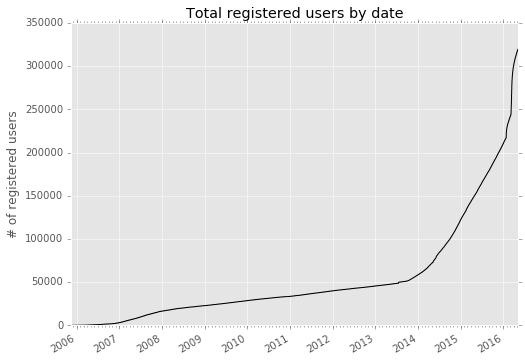After the merge of OGS and Nova, registrations have increased to around 150 per day, currently.

In :
# Registration trends since 2013.  Nova started in January (black line).
# Merge was in October of 2013 (green line).
# The cliff in the curve is a DB-udpate data artifact.
recent = sorted_registered_dates[sorted_registered_dates > datetime64('2012-11')]
base = len(sorted_registered_dates) - len(recent)
plot_time_series(recent, base + arange(len(recent)))
nova_start = datetime64('2013-01-01').astype(datetime.datetime)
ogs_merge = datetime64('2013-10-01').astype(datetime.datetime)
alphago_paper = datetime64('2016-01-28').astype(datetime.datetime)
leesedol_alphago = datetime64('2016-03-09').astype(datetime.datetime)
axvline(nova_start, c='k', lw=0.5)
axvline(ogs_merge, c='g', lw=0.5)
axvline(alphago_paper, c='g', lw=0.5)
axvline(leesedol_alphago, c='g', lw=0.5)

text(nova_start, 90000, " Nova")
text(ogs_merge, 80000, " Merge with OGS")
text(alphago_paper, 80000, "AlphaGo Paper", ha='right')
text(leesedol_alphago, 60000, "Lee Sedol vs. Alphago", ha='right')

ylabel('# of registered users')
title('Same graph as above, since October of 2013')

figure()

recent = sorted_registered_dates[sorted_registered_dates > datetime64('2015-01-01')]
base = len(sorted_registered_dates) - len(recent)
plot_time_series(recent, base + arange(len(recent)))

axvline(alphago_paper, c='g', lw=0.5)
axvline(leesedol_alphago, c='g', lw=0.5)

text(alphago_paper, 150000, "AlphaGo Paper", ha='right')
text(leesedol_alphago, 125000, "Lee Sedol vs. Alphago", ha='right')

ylabel('# of registered users')
title('... and since 2015')
show()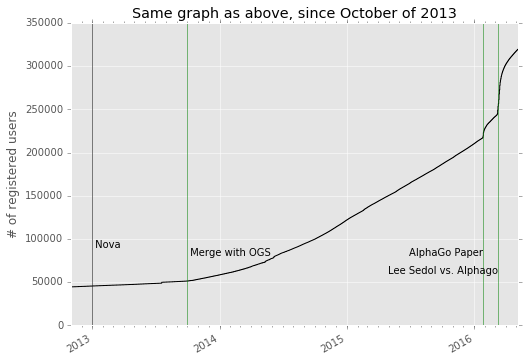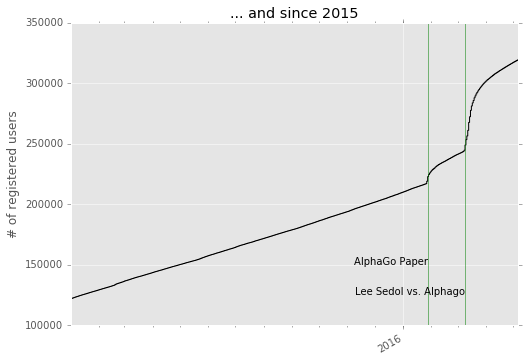Registrations in the last 120 days¶

In :
days_since_registered = ((max(registered_dates) - registered_dates) / timedelta64(1,'D')).astype(int)
hist(days_since_registered[days_since_registered < 120], bins=arange(121))
ylabel('registrations per day')
xlabel('days since {}'.format(max(registered_dates)))
show()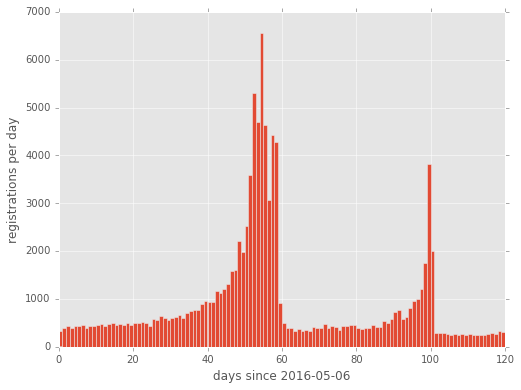Site Activity¶

OGS keeps track of when users connect to the site. last_active stores the timestamp of the last time a user was online.

Unfortunately, some of this data was lost in a structural change in the Redis storage, but it goes back roughly 100 days.

In :
np.array([1, 2, np.inf]).astype('datetime64[s]')
Out:
array(['1970-01-01T00:00:01', '1970-01-01T00:00:02', 'NaT'], dtype='datetime64[s]')
In :
# last_active is in seconds since the epoch.  Some values are "None", represented as infinity, or other weird values
last_day_active = last_active.astype('datetime64[s]')
days_since_active = (max(last_day_active) - last_day_active) / np.timedelta64(1, 'D')
keep = isfinite(last_active) & (days_since_active < 365)
days_since_active = days_since_active[keep]
last_day_active = last_day_active[keep]

print("Number of users: {}".format(len(last_active)))
print("Number without a last_active entry in the last 365 days: {}".format(sum(~keep)))

hist(days_since_active, 200)
ylabel('# of users last seen N days ago')
xlabel('number of days (N)')
axis('tight')

figure()
# January 1 1970 was a Thursday - map this to 4 so Sunday is 0
day_of_week = (((last_day_active - np.datetime64(0, 's')) / np.timedelta64(1, 'D')).astype(int) + 4) % 7

hist(day_of_week[np.logical_and(days_since_active < 365, days_since_active > 27)],
bins=np.linspace(-0.5, 6.5, 8),
normed=True)
title('Day of week')
ylabel('fraction of users last active on this day of the week')
xticks(np.arange(7),
'Sun Mon Tue Wed Thu Fri Sat'.split(' '),
rotation=45)
axis('tight')
show()
Number of users: 319472
Number without a last_active entry in the last 365 days: 137443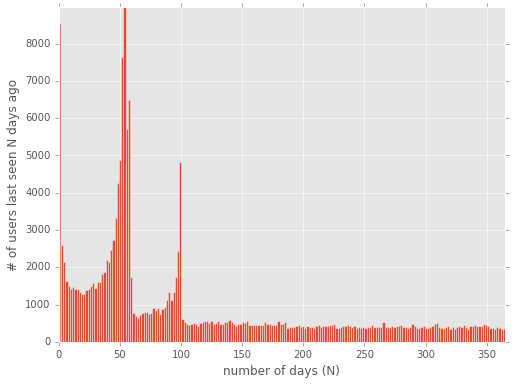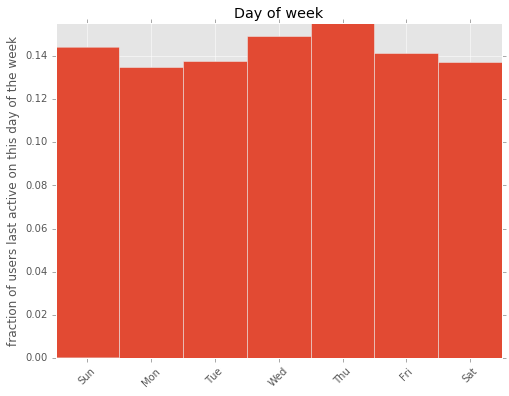Days since active vs days since registered¶

We can look at users' activity vs. when they registered.

In :
days_since_active = (max(last_active) - last_active) / (24 * 60 * 60)
days_since_registered = max(registered_dates) - registered_dates

DATA_BREAKPOINT = 365
keep = days_since_active < DATA_BREAKPOINT
days_since_active = days_since_active[keep]
days_since_registered = days_since_registered[keep] / timedelta64(1,'D')
days_since_registered += np.random.uniform(-0.5, 0.5, days_since_registered.shape)

scatter(days_since_registered, days_since_active, alpha=0.2, lw=0)
xlabel('days since registering')
ylabel('days since last active')
axis('tight')

figure()
xlabel('days since registering')
ylabel('days since last active')
title('Same as above, for last {} days'.format(DATA_BREAKPOINT))
axis('tight')

figure()
DATA_BREAKPOINT = 60
xlabel('days since registering')
ylabel('days since last active')
title('Same as above, for last {} days'.format(DATA_BREAKPOINT))
axis('tight')
Out:
(-3.5284844171777578,
63.020967539242633,
-3.0457189294286753,
63.827856938687681)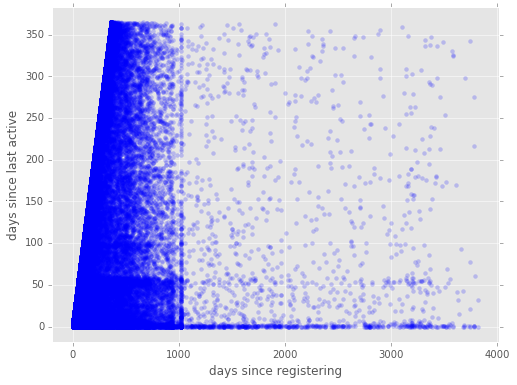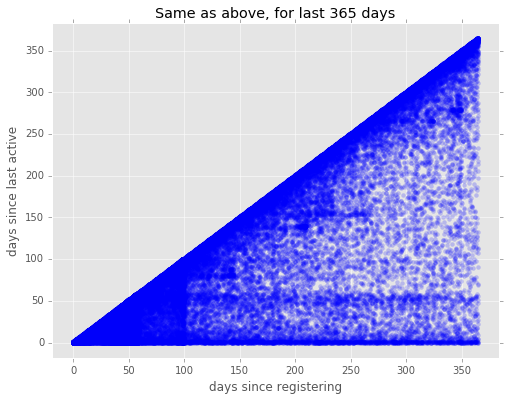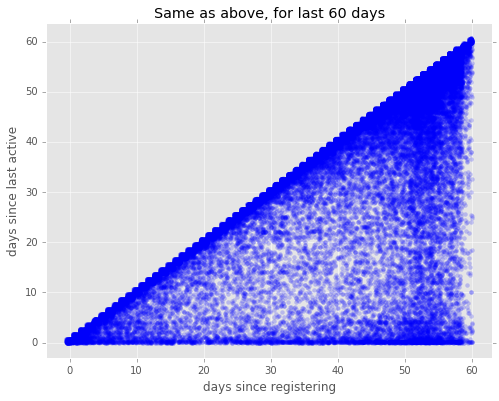It's pretty clear that most users register, stay one or two days, and then don't reappear. Let's look at that more in depth.

What percentage of users stay active, and how has that changed over time?¶

We can look at the number of registrations each day and what percentage of those users were still active. OGS seems to have around 5-10% new registrations result in an active player. It's possible that fraction is increasing as the player base grows, but this will need to be evaluated in the future.

In :
days_since_active = (max(last_active) - last_active) / (24 * 60 * 60)
days_since_registered = ((max(registered_dates) - registered_dates) / timedelta64(1,'D')).astype(int)
DATA_BREAKPOINT = 365
keep = days_since_registered < DATA_BREAKPOINT
days_since_active = days_since_active[keep]
days_since_registered = days_since_registered[keep]
registrations_per_day = bincount(days_since_registered)
active_per_days = bincount(days_since_registered, weights=(days_since_active <= 7))

# smooth to show trend
from scipy.ndimage.filters import gaussian_filter
def smooth(d):
return gaussian_filter(d, 3.5) # half a week
ratio = smooth(active_per_days) / smooth(registrations_per_day)
plot(ratio)
xlabel('days since registration')
ylabel('fraction active within 7 days')
axhline(0.1, c='k')
axhline(0.05, c='b')
yticks(linspace(0, 1.0, 11), ['%02d%%' % (i * 10) for i in range(11)])
axis('tight')
show()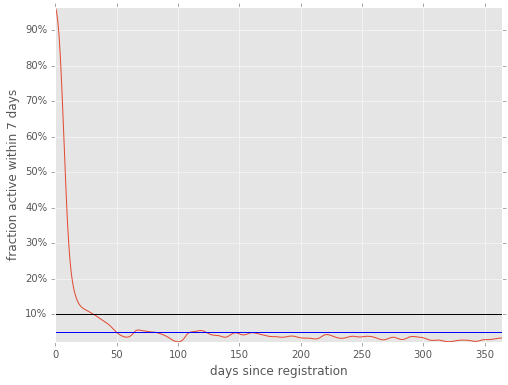Games played vs. registration time or last time active¶

Another measure of activity is the number of ranked games a player has completed.

There are a few (non-computer) players with thousands of games.

In :
print("min, max games:", min(games_count), max(games_count))
no_games = games_count == 0
supers = games_count > 1000
print("# of users with 0 games: {} out of {}".format(sum(no_games), len(games_count)))
print("# of users with more than 1000 games:", sum(supers))

normals = ~ logical_or(no_games, supers)
('min, max games:', 0, 83475)
# of users with 0 games: 182743 out of 319472
('# of users with more than 1000 games:', 830)
In :
hist(games_count[normals], 75, log=True)
title('# of games finished log-scale')
xlabel('number of games')
ylabel('number of users')
figure()

hist(log10(games_count[~ no_games]), 30, log=True)
title('# of games finished log-log-scale')
xticks([0,1,2,3], ["1", "10", "100", "1000"])
axis('tight')
xlabel('number of games')
ylabel('number of users')
show()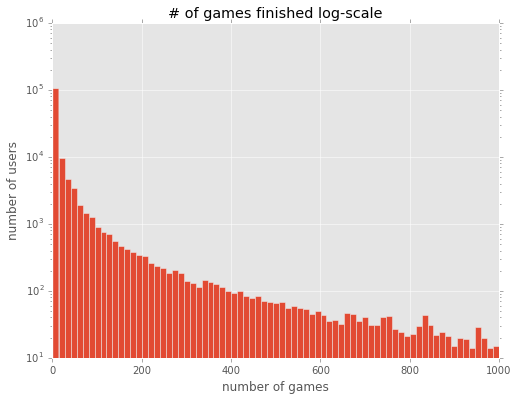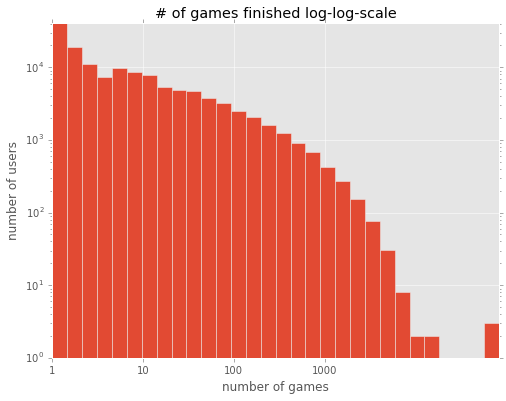Number of games vs last active for players with more than 10 games¶

As expected, completing more games correlates with being more active.

In :
days_since_active = (max(last_active) - last_active) / (24 * 60 * 60)
mask = np.logical_and(games_count > 10, days_since_active < 100)
xticks([0,1,2,3], ["1", "10", "100", "1000"])
axis('tight')
xlabel('number of games completed')
ylabel('days since active')
show()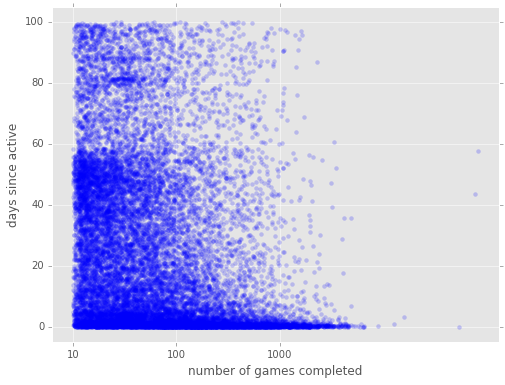Rating vs. Game count or Last active¶

Also, more games tends to correlate with higher ratings, and higher ratings with more active players.

In :
def rating_to_rank(r):
if r >= 2100:
return "{}d".format(1 + (r - 2100) / 100)
else:
return "{}k".format((2100 - r) / 100)

yt = [100,  500, 1000, 1500, 1700, 1900, 2100, 2300, 2500, 2700]
In :
xticks([0,1,2,3], ["1", "10", "100", "1000"])
axis('tight')
xlabel('number of games')
ylabel('rating')
yticks(yt, [rating_to_rank(y) for y in yt])
show()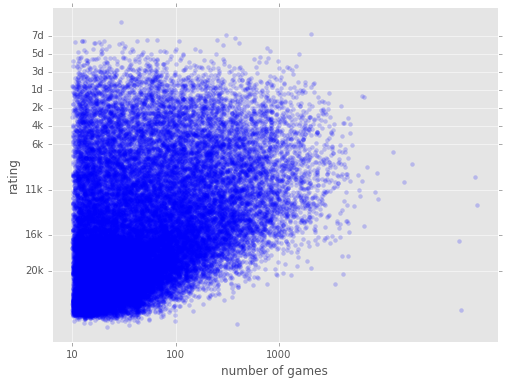In :
days_since_active = (max(last_active) - last_active) / (24 * 60 * 60)
mask = np.logical_and(games_count > 10, days_since_active < 50)